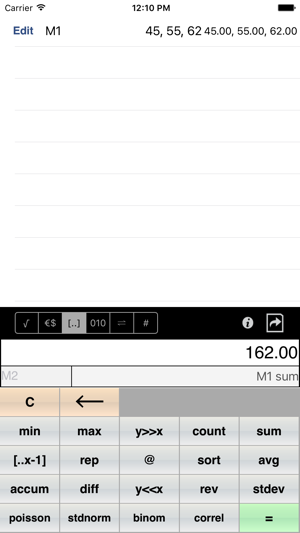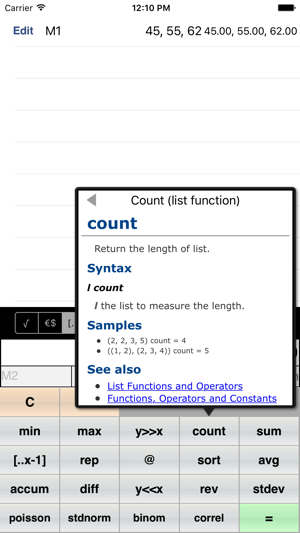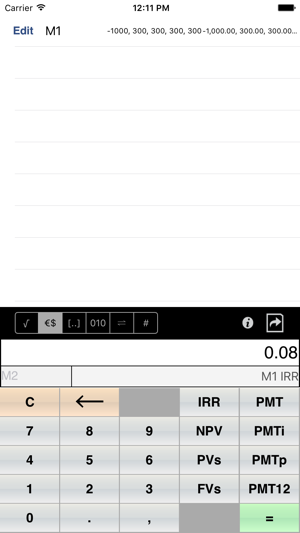## Capturas de tela

•••••## Descrição

neu.Calc is an innovative financial/scientific calculator, which makes complex calculations much easier than traditional calculators or spreadsheet applications.

Also includes unit conversion and a programmer's calculator.

◆ List - a series of numbers

One of the unique features of neu.Calc is the ability to deal with a series of numbers (list) directly.

If you want to calculate the average of a series of numbers, simply input the numbers a list, then tap the "avg" button.

(45, 55, 58, 62, 72, 80, 100) avg = 67.43

If you want to calculate the internal rate of return for a series of cash flows, input those numbers and tap the "IRR" button.

(-1000, 300, 300, 300, 300) IRR = 0.0771

◆ Label and references

Each time the "=" button is used, neu.Calc creates a formula. Each formula is automatically labeled (M1, M2, M3, ..., ) and can be referenced by other formulas. To reference an existing formula, tap on it in the sheet. Labels can be changed by tapping on the label to the left of the formula, when the formula is being entered or edited.

M1: 45, 55, 58, 62, 72, 80, 100 = 45, 55, 58, 62, 72, 80, 100
M2: M1 avg = 67.43
M3: M1 sum = 472.00
M4: M1 stdev = 16.97
M5: 60, 52, 50, 70, 100, 74, 95 = 60, 52, 50, 70, 100, 74, 95
M6: M1 correl M5 = 0.75

Here is another example.

M1: -1000 = -1000
M2: 300 = 300
M3: M1, M2 rep 4 = -1000, 300, 300, 300, 300
M4: 6 % = 0.06
M5: M3 NPV M4 = 39.53

◆ Solver

Solver is a powerful tool to solve linear equations. In order to solve an equation, you need to first create a formula for an unknown value with an estimate, and create a formula (or formulas) that represents the equation.

Here is a simple example to solve the equation "x * 2.5 = 100", where the estimate is 10.

M1: 10 = 10.00
M2: M1 * 2.5 - 100 = -75.00

In order to get the appropriate value for M1 (x in the original equation) that makes the result of M2 zero, tap the "Edit" button of M1, then the "Solve" button of M2. You'll get the following result.

M1: 40.00 = 40.00
M2: M1 * 2.5 - 100 = 0.00

To demonstrate a more complex case, take a look at the NPV example again.

M1: -1000 = -1000
M2: 300 = 300
M3: M1, M2 rep 4 = -1000, 300, 300, 300, 300
M4: 6 % = 0.06
M5: M3 NPV M4 = 39.53

If you want to know the minimum yearly income to justify this investment, tap the "edit" button of M2, and tap the "solve" button of M5. You'll get this.

M1: -1000 = -1000
M2: 288.59 = 288.59
M3: M1, M2 rep 4 = -1000, 288.59, 288.59, 288.59, 288.59
M4: 6 % = 0.06
M5: M3 NPV M4 = 0.00

Versão 1.5

Fixed crash in settings.

## Avaliações e opiniões

4.0 de 5
4 avaliações

4 avaliações

u46 ,

### The best calc over there.

Good for the ones needing a simple calc but also powerful even for those used to RPN.

## Informações

Vendedor
neu.Pen LLC
Tamanho
2.9 MB
Categoria

Requer o iOS 8.0 ou posterior. Compatível com iPhone, iPad e iPod touch.

Idiomas

Inglês

Classificação indicativa
4+
Preço
Grátis

## Compatível com

•